#Interactive Real Analysis

Next | Previous | Glossary | Map

## 7.2. Integration Techniques

### Example 7.2.12(c): Integrating Rational Functions

Find the integral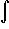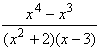dx
This time it seems that we can not use partial fraction decomposition because the degree of the numerator is higher than that of the denominator. To remedy that problem, we use "long division" to divide the polynomials:= x + 2 +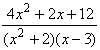Integrating x + 2 is easy, so the problem is reduced to findingdx and the partial fractions decomposition theorem applies just fine to this integrand. We know that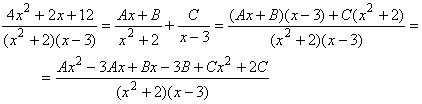Therefore we get three equations in three unknowns:
 -3B + 2C = 12 (for the constant coefficient) -3A + B = 2 (for the x coefficient) A + C = 4 (for the x2 coefficient)
Solving this system of equations gives
A = -10/11, B = -8/11, C = 54/11
Therefore the one complicated integral above changes into three simpler ones: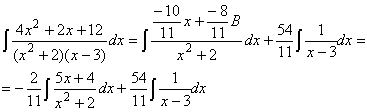The second integral is easy (involving the ln). The first integral seems to be somewhat difficult, because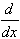arctan(x) = 1 / (1 + x2) which does not quite work, and the numerator is not the derivative of the denominator, so the ln is out, too. But a little algebra and some substitution will do the trick: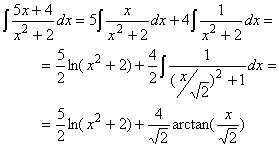Taking everything together gives: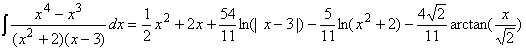Next | Previous | Glossary | Map